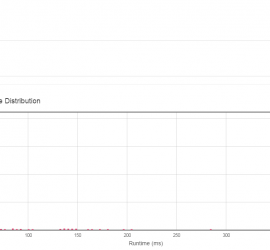# Daily Archives: September 12, 2015

## [leetcode] 3Sum Smaller

3Sum Smaller Given an array of n integers nums and a target, find the number of index triplets i, j, k with 0 <= i < j < k < n that satisfy the condition nums[i] + nums[j] + nums[k] < target. For example, given nums = [-2, 0, 1, […]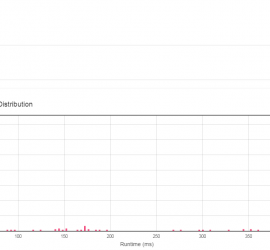## [leetcode] Wiggle Sort

Wiggle Sort Given an unsorted array nums, reorder it in-place such that nums <= nums >= nums <= nums…. For example, given nums = [3, 5, 2, 1, 6, 4], one possible answer is [1, 6, 2, 5, 3, 4]. sort the array, then swap each two adjacent elements. class Solution […]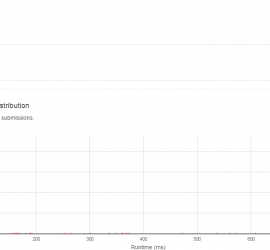## [leetcode] Number of Islands3

Number of Islands Given a 2d grid map of ‘1’s (land) and ‘0’s (water), count the number of islands. An island is surrounded by water and is formed by connecting adjacent lands horizontally or vertically. You may assume all four edges of the grid are all surrounded by water. Example […]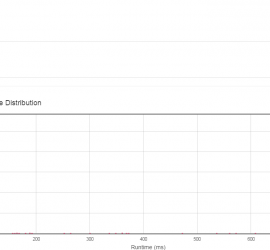## [leetcode] Binary Tree Right Side View

Binary Tree Right Side View Given a binary tree, imagine yourself standing on the right side of it, return the values of the nodes you can see ordered from top to bottom. For example: Given the following binary tree, 1 <— / \ 2 3 <— \ \ 5 4 […]## [leetcode] Number of 1 Bits

Number of 1 Bits Write a function that takes an unsigned integer and returns the number of ’1′ bits it has (also known as the Hamming weight). For example, the 32-bit integer ’11′ has binary representation 00000000000000000000000000001011, so the function should return 3. Credits: Special thanks to @ts for adding […]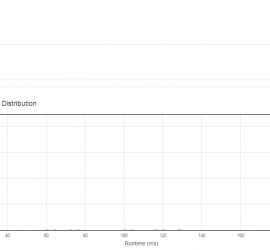## [leetcode] Reverse Bits

Reverse Bits Reverse bits of a given 32 bits unsigned integer. For example, given input 43261596 (represented in binary as 00000010100101000001111010011100), return 964176192 (represented in binary as00111001011110000010100101000000). Follow up: If this function is called many times, how would you optimize it? Related problem: Reverse Integer Credits: Special thanks to @ts […]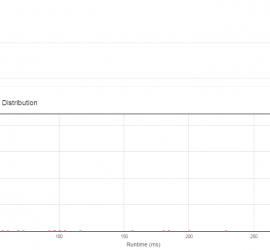## [leetcode] Rotate Array

Rotate Array Rotate an array of n elements to the right by k steps. For example, with n = 7 and k = 3, the array [1,2,3,4,5,6,7] is rotated to [5,6,7,1,2,3,4]. Note: Try to come up as many solutions as you can, there are at least 3 different ways to […]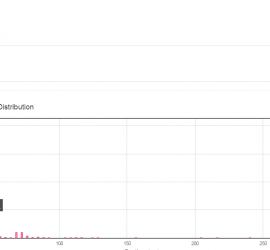## [leetcode] Repeated DNA Sequences

Repeated DNA Sequences All DNA is composed of a series of nucleotides abbreviated as A, C, G, and T, for example: “ACGAATTCCG”. When studying DNA, it is sometimes useful to identify repeated sequences within the DNA. Write a function to find all the 10-letter-long sequences (substrings) that occur more than […]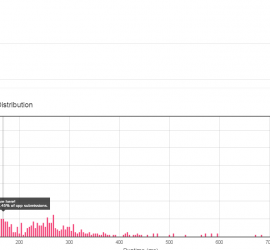## [leetcode] Largest Number

Largest Number Given a list of non negative integers, arrange them such that they form the largest number. For example, given [3, 30, 34, 5, 9], the largest formed number is 9534330. Note: The result may be very large, so you need to return a string instead of an integer. […]